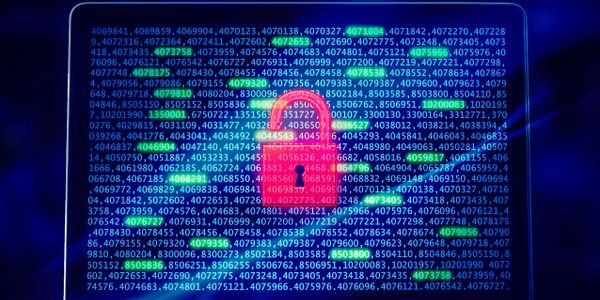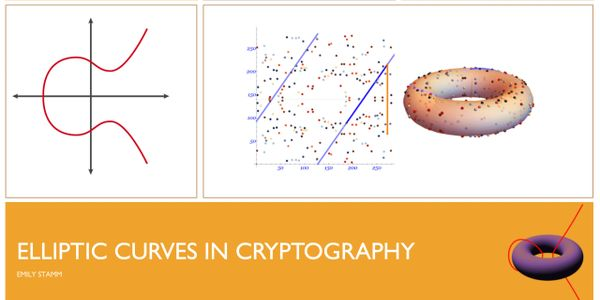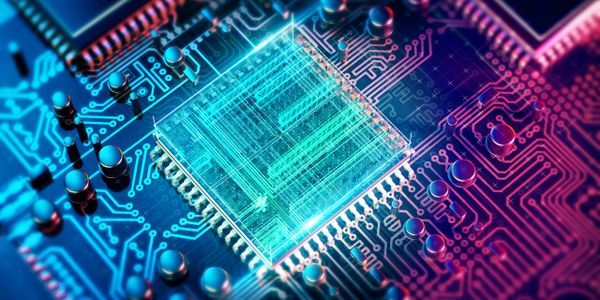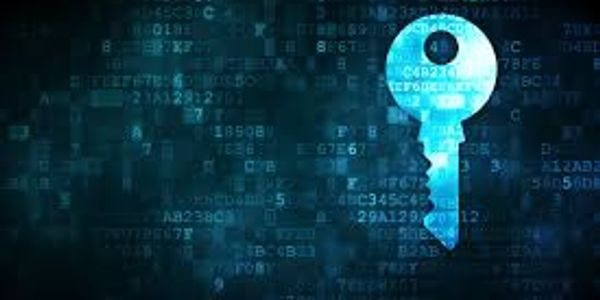#### Elliptic Curves in CryptographyWe explain the effect of quantum computers on public key cryptography. We describe the mathematics of  post-quantum cryptography, including lattice-based, code-based, multivariate, isogeny-based cryptography.

#### Elliptic Curves in CryptographyAn elliptic curve is a type of mathematical curve on a plane. We describe what an elliptic curve is and why it is important in public key cryptography. We explain the classical elliptic curve cryptography (ECC) that is widely used today, as well as the modern post-quantum isogeny-based cryptography.

#### Quantum Game TheoryRSA is the most widely used public key cryptography algorithm, from encryption in HTTPS to digital signatures. We describe RSA encryption and digital signatures algorithms. We demonstrate the Bleichenbacher Million Message attack on RSA and explain how to prevent this attack.  We describe the effect of quantum computers on RSA.

#### Quantum Game TheoryQuantum computing is a new form of computing based on quantum mechanics rather than classical mechanics. Quantum game theory is an emerging field in which quantum algorithms are used to find new solutions to game theory problems. We  demonstrate several games using a Quantum Virtual Machine including a Coin Flip game and the Prisoner’s Dilemma.

#### Lattice CryptographyAdvanced Encryption Standard (AES) is one of the most widely used cryptographic algorithms. We provide an overview of the algorithm, modes of operation, implementations, and security of AES. We demonstrate a simple attack on the AES-ECB mode, and discuss available secure AES modes and implementations.

#### Lattice CryptographyLattice-based cryptography is the most popular form of post-quantum cryptography and the mathematics and design also accommodates encryption, signatures, hash functions, and newer modern cryptographic protocols like identity-based encryption, homomorphic encryption, and functional encryption. In particular, lattice schemes are the only known mathematics currently that allow secure and practical fully homomorphic encryption.

## Public Speaking

#### Presentations

Chicago Quantum Exchange Invited Talk   “Mathematics of Post-Quantum Cryptography & the CyberSecurity Non-Profit” (Webinar 2020)

Seattle CyberSecurity Event  “RSA Cryptography” (Webinar 2020)

Boston CyberSecurity Event on Cryptography “Elliptic Curves in Cryptography” (Boston, MA 2020)

Chicago CyberSecurity Event on Cryptography “Post-Quantum Cryptography” (Chicago, IL 2020)

Illinois State University Colloquium Invited Talk “Post-Quantum Cryptography” (Normal, IL 2019)

Boston CyberSecurity Event on Real-World Encryption “RSA Cryptosystem” (Boston, MA 2019)

Analytic and Combinatorial Number Theory: The Legacy of Ramanujan “An Investigation of (Nearly) Weakly Primes and Sieve Methods” (Urbana, IL 2019)

ForenSecure “Post-Quantum Cryptography: A Survey” (Wheaton, IL 2019)

Women in Cyber Security (IIT) “AES: Advanced Encryption Standard” (Chicago, IL 2019)

Joint Mathematics Meetings “Lacunary Eta-quotients modulo powers of primes” (San Diego, CA 2018)

Southeastern Undergraduate Mathematics Conference“Lacunary Eta-quotients modulo powers of primes” (Atlanta, GA 2017)

Joint Mathematics Meetings “When is a^n+1 the sum of two squares?” (Atlanta, GA 2017)

UGA Mock AMS Conference “When is a^n+1 the sum of two squares?” (Athens, GA 2016)

#### Presentations

P33 Quantum Finance Roundtable  at Chicago Quantum Exchange (Chicago, IL 2020)

Cybersecurity Career Workshop at DePaul University (Chicago, IL 2020)

Careers in Security Panel with CSNP - organizer and moderator (Webinar 2020)